# New Features in the R Graphics Engine

Support for gradient fills, pattern fills, clipping paths and masks has been added to the R graphics engine (in the development version of R, which will probably become R version 4.1.0).

An R-level interface for these new features has been added to the ‘grid’ graphics package.

``library(grid)``

For example, the following code fills a circle with a linear gradient.

``grid.circle(gp=gpar(col=NA, fill=linearGradient()))``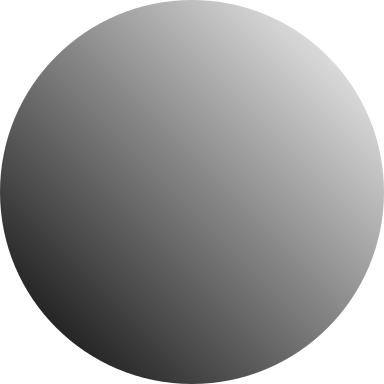The next code fills a rectangle with a radial gradient.

``````grid.rect(gp=gpar(fill=radialGradient(c("white", "black"),
cx1=.8, cy1=.8, r1=0)))``````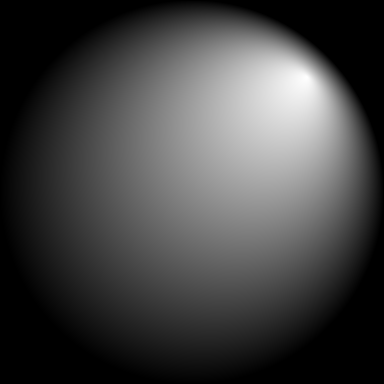The next code fills a rectangle with a pattern of grey diamonds (by repeating a single grey diamond drawn in the centre of the image).

``````pat <- pattern(polygonGrob(unit(.5, "npc") + unit(c(-2, 0, 2, 0), "mm"),
unit(.5, "npc") + unit(c(0, 2, 0, -2), "mm"),
gp=gpar(col=NA, fill="grey")),
width=unit(4, "mm"), height=unit(4, "mm"),
extend="repeat")
grid.rect(gp=gpar(col=NA, fill=pat))``````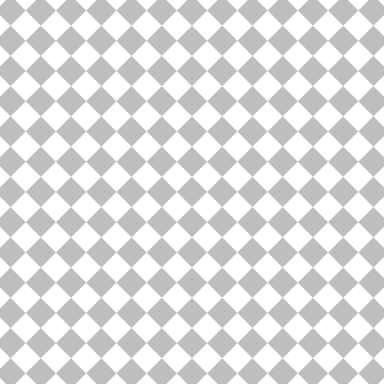The following code applies a mask to the circle with a linear gradient fill. First we show the mask itself, which is a rectangle filled with a linear gradient from black to transparent (drawn over the grey diamond fill to show the transparency).

``````mask <- rectGrob(gp=gpar(col=NA,
x1=.1, y1=.1)))
grid.rect(gp=gpar(col=NA, fill=pat))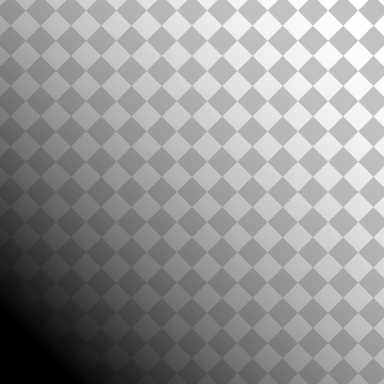Now we apply the mask to the circle with a linear gradient fill, by pushing a viewport with the mask as part of the viewport definition. Again, we draw over the top of the diamond fill to show how the semitransparency of the mask dictates the semitransparency of the circle.

``````grid.rect(gp=gpar(col=NA, fill=pat))
popViewport()``````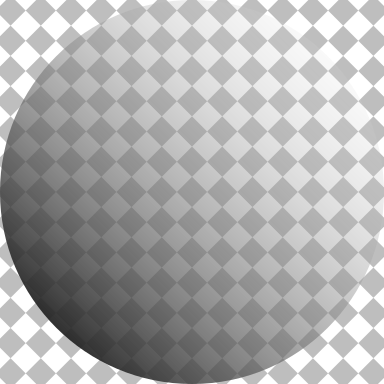Finally, the next code shows a clipping path. We define a circular clipping path by specifying a grob as the `clip` argument for a viewport, then we draw the previous image, which gets clipped to the circular clipping path.

``````pushViewport(viewport(clip=circleGrob()))
grid.rect(gp=gpar(col=NA, fill=pat))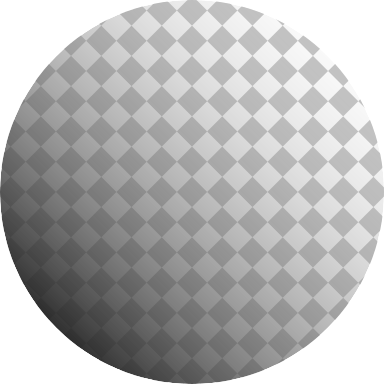The new features have only been implemented on a subset of graphics devices so far: `cairo_pdf()`, `cairo_ps()`, `x11(type="cairo")`, `png(type="cairo")`, `jpeg(type="cairo")`, `tiff(type="cairo")`, `svg()`, and `pdf()`. Although there is no support yet for `quartz()` or `windows()`, almost all of the graphics devices above will work on all major platforms.